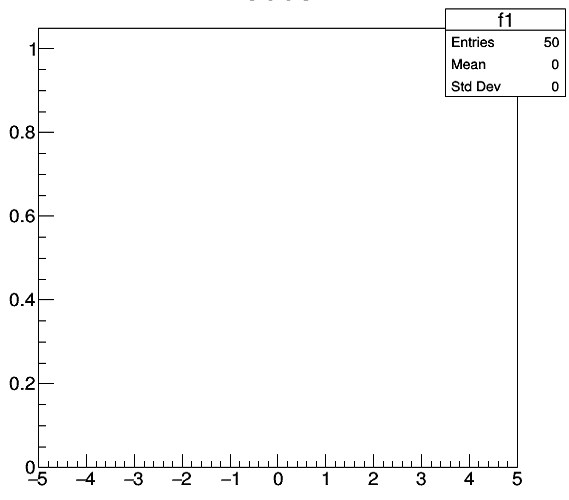# HOW to put an array into a one-dimensional histogram?

I am trying to put an array cs (which is double type) into a one-dimensional histogram, but my code have some problem,and the TH1D is like this. Can you help me to correct my code?
The following is my code:

``````TCanvas *c2=new TCanvas("c2","cs",600,600);
TH1D *h1=new TH1D("h1","cs",50,-5,5);
for(int i=0;i<50;i++)
{
double x =cs[i];
h1->Fill(x);
h1->Draw();
}
``````ROOT Version: Not Provided
Platform: Not Provided
Compiler: Not Provided

Hi,

can you show the content of your array?

1. You shouldn’t start from bin #0, so please replace
``````for(int i=0;i<50;i++)
``````

with

``````for(int i=1;i<=50;i++)
``````
``````h1->Draw();
`for(int i = 0; i < 50; i++) h1->SetBinContent(i + 1, cs[i]);`